# Problems on Decimal - Aptitude test, questions, shortcuts, solved example videos

Video on Decimal - shortcuts, tips and tricks

## Decimal Fractions

Points to Remember

1) Fraction: It represents a part of a whole or more generally, any number of equal parts. If a unit is divided into any number of equal parts, then one or more of these parts is termed as a fraction of the unit.

The numerator in a fraction represents a number of equal parts and denominator (≠0) represents how many of those parts make up a unit or a whole.

2) The value of fraction (x/y) = 1, if numerator = denominator
3) The value of fraction is zero,if numerator=0 and denominator ≠0
4) The value of fraction is infinity,if the denominator=0
5)The value of fraction remains unchanged,even if the numerator and denominator are multiplied or divided by same number
6) Pure recurring decimal: In a decimal fraction, if all the numbers after decimal point repeat, then it is called as pure recurring decimal.
7) Mixed recurring decimal: In a decimal fraction, if some numbers are repetitive and some are not, then it is called as mixed recurring decimal.

Basic Formulae: (Must Remember)
1) (a - b)2 = (a2 + b2 - 2ab)
2) (a + b)2 = (a2 + b2 + 2ab)
3) (a + b) (a – b) = (a2 – b2 )
4) (a3 + b3) = (a + b) (a2 – ab + b2)
5) (a3 - b3) = (a - b) (a2 – ab + b2)
6) (a + b + c)2 = a2 + b2 + c2 + 2 (ab + bc + ca)
7) (a3 + b3 + c3 – 3abc) = (a + b + c) (a2 + b2 + c2 – ab – bc – ac)

Operations on decimal fractions:
Here different operations performed on decimal fractions along with certain examples are given. Clear the concepts point by point and then move further to quick tips and tricks.

Points covered:
1) Addition and subtraction of decimal fractions
2) Multiplication of decimal fractions by power of 10.
3) Multiplication of decimal fractions.
4) Decimal fraction ÷ Counting number
5) Decimal fraction ÷ Decimal fraction

1) Addition and subtraction of decimal fractions
To add or subtract the decimal fractions, numbers are placed under each other in such a way, that the decimal points lie in one column.
Eg: 12.361 + 0.236 + 1236.1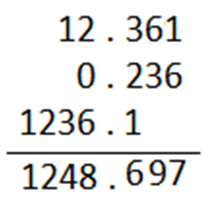2) Multiplication of decimal fractions by power of 10.
The decimal point in the given number is shifted as many places as is the power of 10.
Eg: 4.236 × 100 = 423.6 or 0.563 × 1000 = 563

3) Multiplication of decimal fractions.
Multiply the given numbers without considering the decimal points. Now mark the decimal point in the product by calculating the sum of number of decimal places in the given numbers.

Eg: 0.5 × 0.03 × 0.83
5 × 3 × 83 = 1245
No. of decimal places = 1 + 2 + 2 = 5
Now mark the decimal point after 5 places starting from left.

4) Division of a decimal fraction
Divide the given numbers without considering the decimal points. Now mark the decimal point in the quotient obtained, by calculating the number of decimal places in the dividend.
Eg: (0.0133 ÷ 19)
(133 ÷ 19)=7
Dividend contains 4 places of decimal. Hence, the answer is 0.0007

5) Decimal fraction ÷ Decimal fraction
Eg: Divide 0.00025 by 0.5
Convert 0.5 without decimal place i.e. 5. Multiply numerator and denominator by 10.
Therefore, we get
=0.0025 ÷ 5
=0.0005

Comparison of fractions
 If fraction in the form 4 , 7 5 8
are to be compared or are to be arranged in ascending of descending order,Then simply convert these fractions into decimal form and then compare.
 Eg: Arrange 4 , 3 , 6 in descending order 5 5 8
4/5 = 0.8, 3/5 = 0.6, 6/8 = 0.75

0.8 > 0.75 > 0.6, hence (4/5) > (6/8) > (3/5)

Concept of Pure Recurring Decimal
If in a decimal fraction all the numbers after decimal point repeat, then it is called as pure recurring decimal.

In decimal fraction 23.6666, 6666 are repeating and hence the fraction is called as recurring decimal.

How to convert a Pure Recurring Decimal into Vulgar Fractions?

In a decimal fraction, if there are n numbers of repeated numbers after a decimal point, then just write one repeated number in the numerator and in denominator take n number of nines equal to repeated numbers you observe after the decimal point.

Let’s see an example, to easily understand the conversion
 Example: 0.8 = 8 9

0.8 =0.8888…,this means only one number repeats.

As discussed, numerator is taken as 8 which is the single repeated number after the decimal point and denominator is taken as 9, because there is only one number which repeats after decimal point.
 Example: 0.57 = 57 99
0.57 =0.575757…..,this means 57 is a set of number which repeats
Numerator is taken as 57 (one repeated set) and denominator is taken as 99, (because two numbers 5 and 7 repeat after the decimal point)

How to convert Mixed Recurring Decimal into Vulgar Fraction?

REMEMBER:
Numerator = (All digits after decimal point) Repeated digits only once – (Non-repetitive digit after decimal point)

Denominator = Take 9 as many times the repetitive digit, followed by zeros equal to number of non-repetitive digits.

Check whether you have got the correct answer, by dividing the numerator by denominator of the obtained vulgar fraction.

Example: 0.23434
This is an example of mixed recurring decimal, because after the decimal point number 2 does not repeat and numbers 3 and 4 repeat. This type of number can be converted into fraction as follows:

0.23434… =0.234
 = 234 – 2 = 232 990 990

 0.234 = conversion of mixed recurring decimal into vulgar fraction = 232 990

Question Variety

Type1: Find the fractions in ascending or descending order

Q 1. Which of the following has fractions in ascending order?
 a. 2 , 3 , 1 , 4 , 5 5 5 3 7 6
 b. 1 , 2 , 3 , 5 , 4 3 5 5 6 7
 c. 1 , 2 , 5 , 4 , 3 3 5 6 7 5
 d. 1 , 2 , 4 , 3 , 5 3 5 7 5 6
View solution

Correct Option: (d)

Convert fractions into decimal form. These calculations are to be solved quickly in mind otherwise will require a lot time.
Option 1: 0.4, 0.6, 0.33, 0.5, 0.8 --- (wrong)
Option 2: 0.3, 0.4, 0.6, 0.8, 0.5 --- (wrong)
Option 3: 0.3, 0.4, 0.8 , 0.5, 0.6 --- (wrong)
Option 4: 0.33, 0.4, 0.5, 0.6, 0.8 --- (correct)
0.33 < 0.4 < 0.5 < 0.6 < 0.8 ---- (Ascending order)

Q 2. Which of the following has fractions in descending order?
 a. 5 , 4 , 2 , 3 , 1 6 7 5 5 3
 b. 5 , 3 , 4 , 2 , 1 6 5 7 5 3
 c. 4 , 1 , 2 , 5 , 3 7 3 5 6 5
 d. 1 , 2 , 4 , 3 , 5 3 5 7 5 6
View solution

Correct Option: (b)

Convert fractions into decimal form. These calculations are to be solved quickly in mind otherwise will require a lot time.
Option 1: 0.8, 0.5, 0.4, 0.6, 0.33--- (wrong)
Option 2: 0.8, 0.6, 0.5, 0.4, 0.3 --- (correct)
Option 3: 0.5, 0.3, 0.4 , 0.8, 0.6 --- (wrong)
Option 4: 0.33, 0.4, 0.5, 0.6, 0.8 --- (wrong)
0.8 > 0.6 > 0.5 > 0.4 > 0.3 ---- (Descending order)

Type 2: Convert decimal numbers into vulgar fractions

Q 3. Convert 0.737373… into vulgar fraction?
 a. 73 99
 b. 73 100
 c. 73 90
 d. 73 900
View solution

Correct Option: (a)

Hint: In a decimal fraction, if there are n numbers of repeated numbers after a decimal point, then just write one repeated number in the numerator and in denominator take n number of nines equal to repeated numbers you observe after the decimal point.
0.737373… is written as 0.73
Numerator = 73 ---- (one repeated number)
Denominator = 99 ---- (73 is the number which is repeated)

 Vulgar fraction = 73 99

Q 4. Convert 0.67 into vulgar fraction.
 a. 67 99
 b. 67 90
 c. 61 90
 d. 61 100
View solution

Correct Option: (c)

Hint:
Numerator = (All digits after decimal point) Repeated digits only once – (Non-repetitive digit after decimal point)
Denominator = Take 9 as many times the repetitive digit, followed by zeros equal to number of non-repetitive digits.

0.67 is a mixed recurring fraction.

 Numerator = 67 – 6 = 61 90 90

Q 5. Find the correct expression for 5.46 in the fractional form.
 a. 541 100
 b. 541 99
 c. 546 99
 d. 541 900
View solution

Correct Option: (b)

 5.46 = 5 + 0.46 = 5 + 46 = 495 + 46 = 541 99 99 99
Convert 0.46 into fraction and then add 5 to the fraction obtained.

Type 3: Adding/Subtracting/Dividing decimal fractions

Q 6. 0.2343 + 0.1888 = ?

a. 0.4232
b. 0.4132
c. 0.4233
d. 0.4231
View solution

Correct Option: (a)

0.2343 + 0.1888 are mixed recurring decimal

 0.2343 = 2343 – 23 = 2320 9900 9900
 0.1888 = 1888 – 18 = 1870 9900 9900

0.2343 + 0.1888
 2320 + 1870 = 2320 + 1870 = 4190 = 0.4232 9900 9900 9900 9900

Q 7. 3.23 – 2.03 + 1.55

a. 2.75
b. 2.75
c. 2. 70
d. 2.71
View solution

Correct Option: (b)

3.23 – 2.03 + 1.55
Step 1: (3 + 0.23) + (1 + 0.55) – (2 + 0.33)
Step 2: [4 + (0.23 + 0.55)] – (2 + 0.03)
Step 3: Convert decimal numbers into vulgar fractions

 0.23 = 23 99
 0.55 = 55 99
 0.03 = 9 99
Step 4: Substituting the values, we get
 =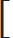4 +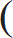23 + 55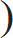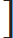–2 + 399 99 99
 =2 +23 + 55 – 399 99 99
 =2 + 7599
= 2.75

Q 8. Find the product of 0.09 × 7.3

a. 0.67
b. 0.657
c. 0.6
d. None of the above
View solution

Correct Option: (c)

 0.09 = 9 99
 7.3 = 7 + 3 = 66 9 9
 9 × 66 = 2 = 6 99 9 3 9
 6 can be easily converted into decimal form 9
= 0.6

 Q 9. If 347.624 = a, then find the value of 347624 = ? 0.0089 0.0089

 a. a 10
b. 10 a
c. a/1000
d. 1000a
View solution

Correct Option: (c)

 Given: 347.624 = a 0.0089
 The value of 347624 ÷ 1000 = a ÷ 1000 = a/1000 0.0089

 Q 10. Find the value of (.555 × .555 – .555 × .020 + .020 × .020) (.555 × .555 × .555) + (.020 × .020 × .020)

a. 1.55
b. 1.74
c. 2.36
d. 5.02
View solution

Correct Option: (b)

 The given numerical is in the form (a2 – ab + b2) = (a2 – ab + b2) = 1 (a3 + b3) (a + b) (a2 – ab + b2) (a + b)

 (0.5552) – (0.555 × 0.020) + (0.202) = 1 = 1.74 (0.5553) + 0.0203 (0.555 + 0.020)

This type of numerical can be easily solved, if all basic formulae are known.

Practice questions on Decimal
Problems on Fraction - Aptitude test, questions, shortcuts, solved example videos
Problems on Fraction - Quantitative aptitude tutorial with easy tricks, tips & short cuts explaining the concepts of the chapter. Online aptitude preparation material with practice question bank, examples, solutions and explanations. Video lectures to prepare quantitative aptitude for placement tests & competitive exams like MBA, Bank exams, RBI, IBPS, SSC, SBI, RRB, Railway, LIC, MAT. Very useful for freshers, engineers & software developers taking entrance exams. Learn and take practice tests!
Problems on Simplification - Aptitude test, questions, shortcuts, solved example videos
Problems on Simplification - Quantitative aptitude tutorial with easy tricks, tips & short cuts explaining the concepts. Online aptitude preparation material with practice question bank, examples, solutions and explanations. Video lectures to prepare quantitative aptitude for placement tests & competitive exams like MBA, Bank exams, RBI, IBPS, SSC, SBI, RRB, Railway, LIC, MAT. Very useful for freshers, engineers & software developers taking entrance exams. Learn and take practice tests!
Square Root and Cube Root - Aptitude test, questions, shortcuts, solved example videos
Square root & Cube root - Quantitative aptitude tutorial with easy tricks, tips & short cuts explaining the concepts. Online aptitude preparation material with practice question bank, examples, solutions and explanations. Video lectures to prepare quantitative aptitude for placement tests & competitive exams like MBA, Bank exams, RBI, IBPS, SSC, SBI, RRB, Railway, LIC, MAT. Very useful for freshers, engineers & software developers taking entrance exams. Learn and take practice tests!